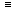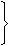# Aptitude - Chain Rule

### Exercise :: Chain Rule - General Questions

11.

A flagstaff 17.5 m high casts a shadow of length 40.25 m. The height of the building, which casts a shadow of length 28.75 m under similar conditions will be:

 A. 10 m B. 12.5 m C. 17.5 m D. 21.25 m

Explanation:

Let the height of the building x metres.

Less lengthy shadow, Less in the height (Direct Proportion)40.25 : 28.75 :: 17.5 : x40.25 x x = 28.75 x 17.5

 x = 28.75 x 17.5 40.25x = 12.5

12.

In a camp, there is a meal for 120 men or 200 children. If 150 children have taken the meal, how many men will be catered to with remaining meal?

 A. 20 B. 30 C. 40 D. 50

Explanation:

There is a meal for 200 children. 150 children have taken the meal.

Remaining meal is to be catered to 50 children.

Now, 200 children120 men.

 50 children120 x 50= 30 men. 200

13.

An industrial loom weaves 0.128 metres of cloth every second. Approximately, how many seconds will it take for the loom to weave 25 metres of cloth?

 A. 178 B. 195 C. 204 D. 488

Explanation:

Le the required time be x seconds.

More metres, More time (Direct Proportion)0.128 : 25 :: 1 : x0.128x = 25 x 1

 x = 25 = 25 x 1000 0.128 128x = 195.31.Required time = 195 sec (approximately).

14.

36 men can complete a piece of work in 18 days. In how many days will 27 men complete the same work?

 A. 12 B. 18 C. 22 D. 24 E. None of these

Explanation:

Let the required number of days be x.

Less men, More days (Indirect Proportion)27 : 36 :: 18 : x27 x x = 36 x 18x = 36 x 18 27x = 24.

15.

4 mat-weavers can weave 4 mats in 4 days. At the same rate, how many mats would be woven by 8 mat-weavers in 8 days?

 A. 4 B. 8 C. 12 D. 16

Explanation:

Let the required number of bottles be x.

More weavers, More mats (Direct Proportion)

More days, More mats (Direct Proportion)

 Wavers 4 : 8:: 4 : x Days 4 : 84 x 4 x x = 8 x 8 x 4x = (8 x 8 x 4) (4 x 4)x = 16.